# Lecture 1

## C

• Today we’ll learn a new language, C: a programming language that has all the features of Scratch and more, but perhaps a little less friendly since it’s purely in text.
• By the end of the term, our goal is not to have learned a specific programming language, but how to program.
• The syntax, or rules around structure, punctuation, and symbols in code, will become familiar to us, even if we might not understand what everything does right away.
• With Scratch, we explored some ideas of programming, like:
• functions
• arguments, return values
• conditionals
• Boolean expressions
• loops
• variables
• Today, we’ll translate some of those ideas to C, a computer language with new syntax and more precision, though fewer words to learn than a human language might include.
• As a first-year student, we might not have known all the information about our new campus right away, but instead learned what we needed to on a day-by-day basis. Here too, we’ll start with the most important details, and “wave our hands” at some of the other details we don’t need quite yet.
• When we evaluate the quality of our code, we might consider the following aspects:
• correctness, or whether our code solves our problem correctly
• design, or how well-written our code is, based on how efficient and readable it is
• style, or how well-formatted our code is visually
• Our first program in C that simply prints “hello, world” to the screen looks like this:
#include <stdio.h>

int main(void)
{
printf("hello, world\n");
}


## IDEs, compilers, interfaces

• In order to turn this code into a program that our computer can actually run, we need to first translate it to binary, or zeroes and ones.
• Tools called IDEs, integrated development environments, will include features for us to write, translate, and run our code.
• One popular IDE, Visual Studio Code, contains a text editor, or area where we can write our code in plain text and save it to a file: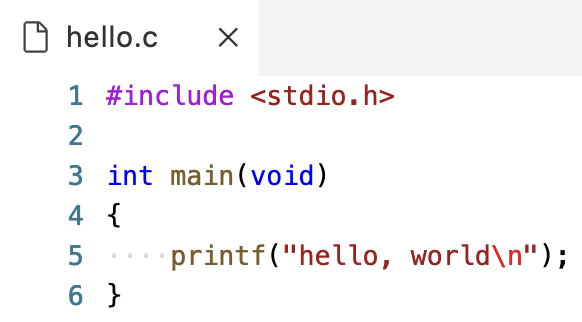• Now our source code, or code that we can read and write, is saved to a file called hello.c. Next, we need to convert it to machine code, or zeroes and ones that represent instructions that tell our computer to perform low-level operations.
• A compiler is a program that can convert one language to another, such as source code to machine code: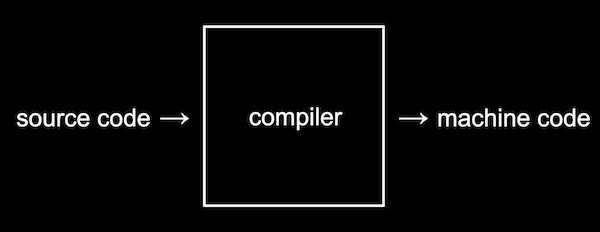• Visual Studio Code, also referred to as VS Code, is typically a program that we can download to our own Mac or PC. But since we all have different systems, it’s easier to get started with a cloud-based version of VS Code that we can access with just a browser.
• In Problem Set 1, we’ll learn how to access our own instance of VS Code.
• In the bottom half of the VS Code interface, we see a terminal, a window into which we can type and run text commands: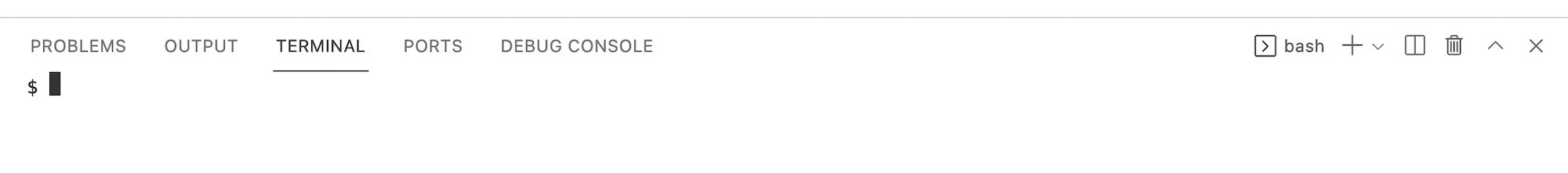• This terminal will be connected to our own virtual server, with its own operating system, set of files, and other installed programs that we access through the browser.
• The terminal provides a command-line interface, or CLI, and it allows us to access the virtual server’s operating system, Linux.
• We’ll run a command to compile our program, make hello. Nothing appears to happen, but we’ll now have another file that’s just called hello, which we can run with ./hello: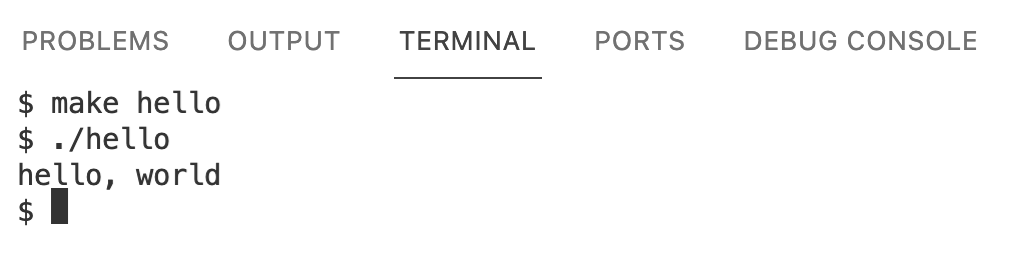• ./hello tells our computer to find a file in our current folder (.), called hello, and run it. And we indeed see the output that we expected.
• We’ll open the sidebar and see that there are two files in our virtual server, one called hello.c (which we have open in our editor), and one called hello: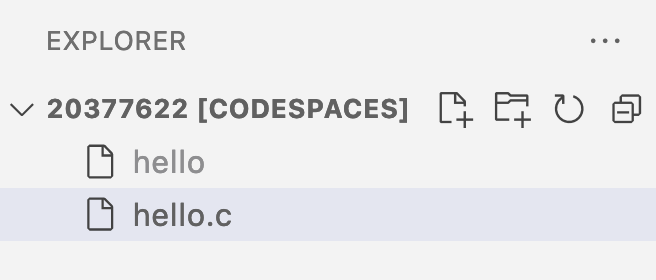• The make hello command created the hello file containing machine code.
• The sidebar is a graphical user interface, or GUI, with which we can interact visually as we typically do.
• To delete a file, for example, we can right-click it in the sidebar and select the “Delete Permanently” option, but we can also use the terminal with the rm command: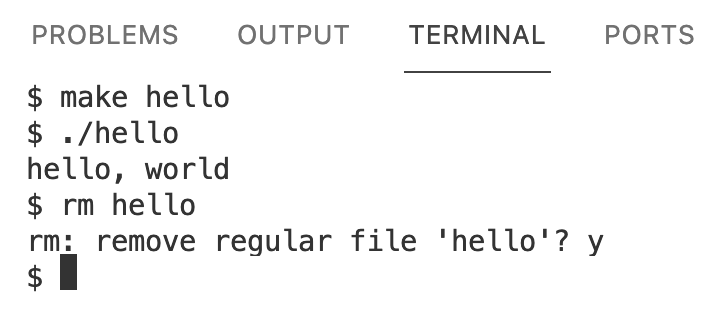• We run rm hello to remove the file called hello, and respond y for “yes” to confirm when prompted.
• We can also run the ls command to list files in our current folder. We’ll compile our file again and run ls to see that a file called hello was created: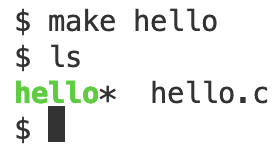• hello is in green with an asterisk, *, to indicate that it’s executable, or that we can run it.
• Now, if we change our source code to read a different message, and run our program with ./hello, we won’t see the changes we made. We need to compile our code again, in order to create a new version of hello with machine code that we can run and see our changes in.
• make is actually a program that finds and uses a compiler to create programs from our source code, and automatically names our program based on the name of the source code’s file.

## Functions, arguments, return values, variables

• Last time, we learned about functions, or actions, and arguments, or inputs to those functions that change what they do.
• The “say” block, for example, is closest to printf in C:
say [hello, world]

printf("hello, world");

• The f in printf refers to a “formatted” string, which we’ll see again soon. And a string is a number of characters or words that we want to treat as text. In C, we need to surround strings with double quotes, "".
• The parentheses, (), allow us to give an argument, or input, to our printf function.
• Finally, we need a semicolon, ;, to indicate the end of our statement or line of code.
• One type of output for a function is a side effect, or change that we can observe (like printing to the screen or playing a sound):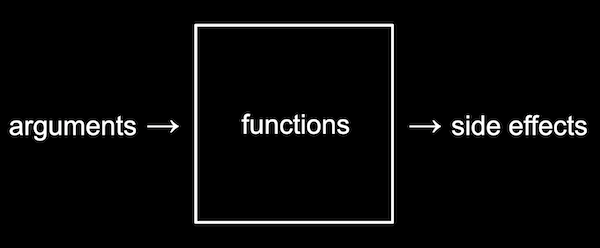• In Scratch, the “say” block had a side effect: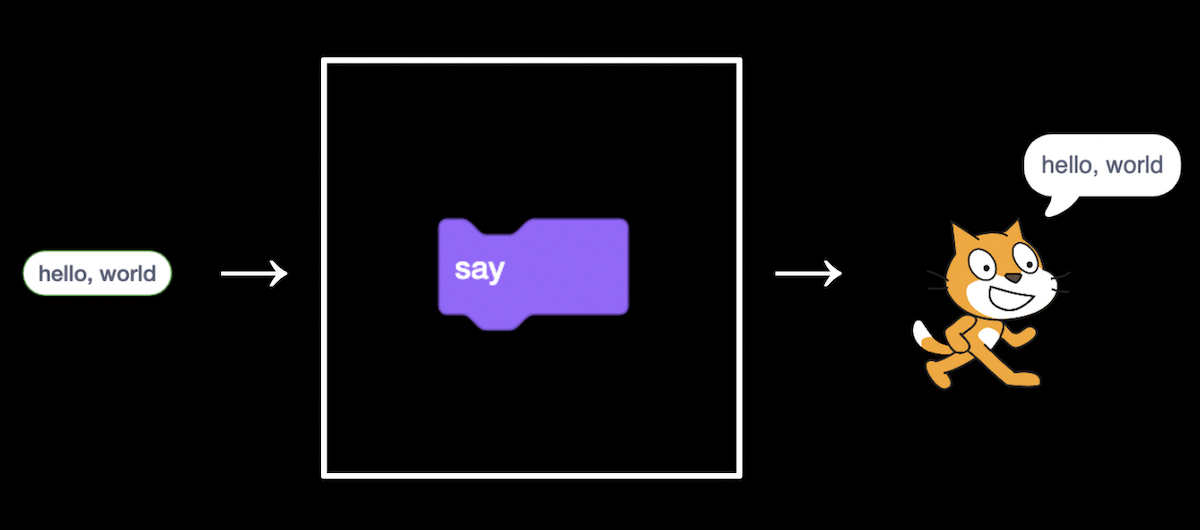• In contrast to side effects, we also saw blocks, or functions, with return values that we can use in our program. That return value might then be saved into a variable.
ask [What's your name?] and wait

string answer = get_string("What's your name? ");

• In C, we have a function called get_string(), into which we pass the argument "What's your name? " as the prompt.
• Then, we save the return value into a variable with answer = . Here, we’re not asking whether the two sides are equal, but rather using = as the assignment operator to set the left side to the value on the right.
• Finally, we need to indicate in C that answer is a variable with the type of string. Another type, int, is short for integer, or whole number. We’ll see other types soon, but this is how our program will interpret different bytes.
• If we try to set a value with a different type to a variable, the compiler will give us an error.
• And just like learning a new human language, it might take weeks or months before we start automatically noticing these small details, like the semicolon. For some programming languages, the convention is to use all lowercase letters for variable and function names, but for others the conventional style might be different.
• We’ll experiment again with our original program, this time removing the \n from the string we pass into printf:
#include <stdio.h>

int main(void)
{
printf("hello, world");
}

• And now, when we compile and run our program, we won’t have the new line at the end of our message:
$make hello$ ./hello
hello, world$ • Let’s try adding a new line within our string: #include <stdio.h> int main(void) { printf("hello, world "); }  • Our compiler will gives us back many errors: $ make hello
hello.c:5:12: error: missing terminating '"' character [-Werror,-Winvalid-pp-token]
printf("hello, world
^
hello.c:5:12: error: expected expression
hello.c:6:5: error: missing terminating '"' character [-Werror,-Winvalid-pp-token]
");
^
hello.c:7:2: error: expected '}'
}
^
hello.c:4:1: note: to match this '{'
{
^
4 errors generated.
make: *** [<builtin>: hello] Error 1

• Since many of these tools like compilers were originally written years ago, their error messages are concise and not as user-friendly as we’d like, but in this case it looks like we need to close our string with a " on the same line.
• When we use \n to create a new line, we’re using an escape sequence, or a way to indicate a different expression within our string. In C, escape sequences start with a backslash, \.
• Now, let’s try writing a program to get a string from the user:
#include <stdio.h>

int main(void)
{
}

• We’ll add a space instead of a new line after “What’s your name?” so the user can type in their name on the same line.
• When we compile this with make hello, we get a lot of errors. We’ll scroll up and focus on just the first error:
$make hello hello.c:5:5: error: use of undeclared identifier 'string'; did you mean 'stdin'? string answer = get_string("What's your name? "); ^~~~~~ stdin /usr/include/stdio.h:137:14: note: 'stdin' declared here extern FILE *stdin; /* Standard input stream. */ ^  • hello.c:5:5 indicates that the error was found on line 5, character 5. It looks like string isn’t defined. • It turns out that, in order to use certain features or functions that don’t come with C, we need to load libraries. A library is a common set of code, like extensions in Scratch, that we can reuse, and stdio.h refers to a library for standard input and output functions. With the line #include <stdio.h>, we’re loading this library that contains printf, so that we can print to the screen. • We’ll need to also include cs50.h, a library written by CS50’s staff, with helpful functions and definitions like string and get_string. • We’ll update our code to load the library … #include <cs50.h> #include <stdio.h> int main(void) { string answer = get_string("What's your name? "); printf("hello, answer\n"); }  • … and now our compiler works. But when we run our program, we see hello, answer printed literally: $ make hello
$./hello What's your name? David hello, answer$

• It turns out, we need to use a bit more syntax:
printf("hello, %s\n", answer);

• With %s, we’re adding a placeholder for printf to format our string. Then, outside our string, we pass in the variable as another argument with answer, separating it from the first argument with a comma, ,.
• Text editors for programming languages will helpfully highlight, or color-code, different types of ideas in our code: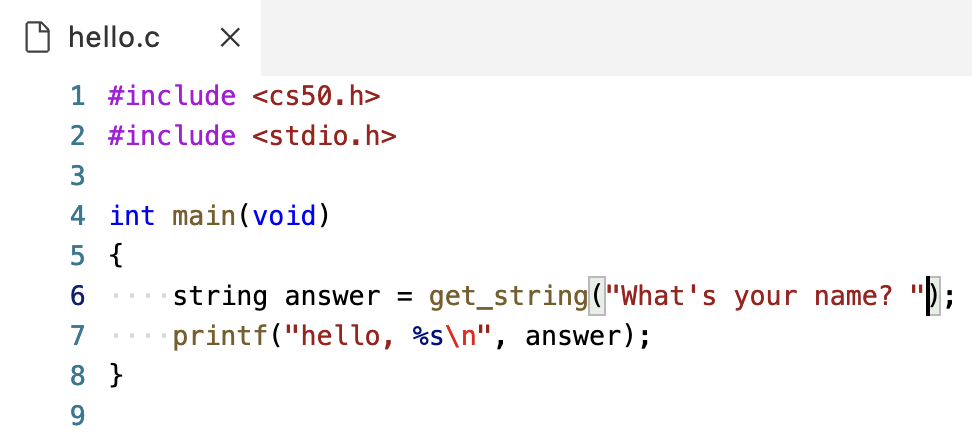• Now, it’s easier for us to see the different components of our code and notice when we make a mistake.
• Notice that on line 6, too, when our cursor is next to a parenthesis, the matching one is highlighted as well.
• The four dots on lines 6 and 7 also help us see the number of spaces for indentation, helping us line up our code.
• We could also use the return value from get_string directly as an argument, as we might have done in Scratch with nested blocks:
#include <cs50.h>
#include <stdio.h>

int main(void)
{
printf("hello, %s\n", get_string("What's your name? ");
}

• But we might consider this to be harder to read, and we aren’t able to reuse the return value later.
• Both get_string in C and the “ask” block in Scratch are functions that have a return value as output: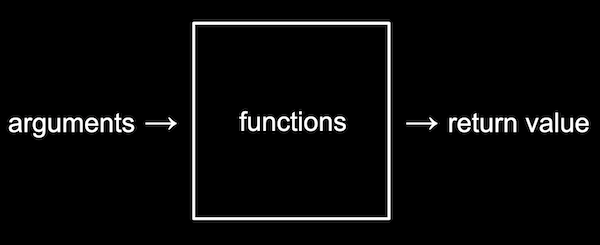• printf("hello, %s\n", answer); is also similar to these Scratch blocks:
say (join [hello,] (answer))

• We’re placing a variable into our string, and displaying it right away.

• In C, main achieves a similar effect as the Scratch block “when green flag clicked”:
when green flag clicked

int main(void)
{

}

• The curly braces, { and }, surround the code that will run when our program is run as well.
• Header files, like stdio.h, tells our compiler which libraries to load into our program. stdio.h is like a menu of functions and features like printf that we can use in our code, though header files themselves don’t include the actual implementation.
• In Linux, there are a number of commands we might use:
• cd, for changing our current directory (folder)
• cp, for copying files and directories
• ls, for listing files in a directory
• mkdir, for making a directory
• mv, for moving (renaming) files and directories
• rm, for removing (deleting) files
• rmdir, for removing (deleting) directories
• In our cloud-based IDE, we’re able to create new files and folders with the GUI in the sidebar:• We can also use the terminal with:
$mkdir pset1$ mkdir pset2
$ls hello* hello.c pset1/ pset2/$

• We’ll run mkdir twice, giving it the names of two folders we want to create. Then, we can run ls to see that our current directory has those folders.
• Now, we can run cd to change our current directory:
$cd pset1/ pset1/$ ls
pset1/ $ • Notice that our prompt, $, changes to pset1/ $ to remind us where we are. And ls shows that our current directory, now pset1, is empty. • We can make yet another directory and change into it: pset1/$ mkdir mario
pset1/ $ls mario/ pset1/$ cd mario/
pset1/mario/ $ • We’ll run a command specific to VS Code, code mario.c, to create a new file called mario.c. We see that it opens in the editor, and we can see our new folders and file in the sidebar as well: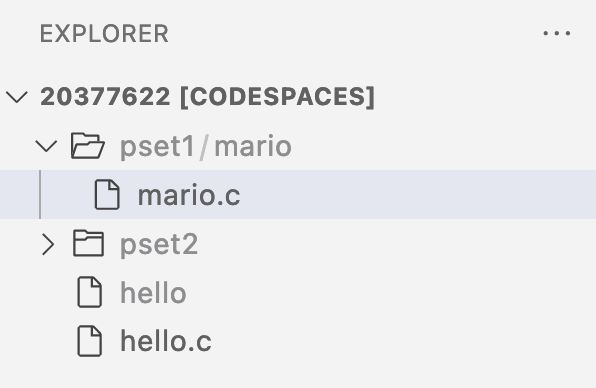• To change our current directory to the parent directory, we can run cd ... We can go up two levels at once with cd ../.. as well: pset1/mario/$ cd ..
pset1/ $cd mario/ pset1/mario/$ cd ../..
$ • cd on its own will also bring us back to our default directory: pset1/mario/$  cd
$ • And . refers to the current directory, which allows us to run a program hello in our current directory with ./hello. ## Types, format codes, operators • There are many data types we can use for our variables, which indicate to our program what type of data they represent: • bool, a Boolean expression of either true or false • char, a single character like a or 2 • double, a floating-point value with more digits than a float • float, a floating-point value, or real number with a decimal value • int, integers up to a certain size, or number of bits • long, integers with more bits, so they can count higher than an int • string, a string of characters • And the CS50 Library has corresponding functions to get input of various types: • get_char • get_double • get_float • get_int • get_long • get_string • For printf, too, there are different placeholders for each type, called format codes: • %c for chars • %f for floats or doubles • %i for ints • %li for long integers • %s for strings • There are several mathematical operators we can use, too: • + for addition • - for subtraction • * for multiplication • / for division • % for remainder ## Variables, syntactic sugar • We might create a variable called counter and set its value to 0 in Scratch and C with the following: set [counter v] to (0)  int counter = 0;  • And we can increase the value with: change [counter v] by (1)  counter = counter + 1;  • In C, we’re taking the original value of counter, adding 1, and then assigning it into the left side, or updating the value of counter. • We don’t need to specify the type of counter again, since it’s been created already. • C also supports syntactic sugar, or shorthand expressions for the same functionality. We could equivalently say counter += 1; to add one to counter before storing it again. We could also just write counter++;, or even counter--; to subtract one. ## Calculations • Let’s create a new file in our instance of VS Code with the command code calculator.c in our terminal. Then, we’ll add in the following code to the editor that’s opened for us, and save the file: #include <cs50.h> #include <stdio.h> int main(void) { int x = get_int("x: "); int y = get_int("y: "); printf("%i\n", x + y); }  • We’ll prompt the user for two variables, x and y, and print out the sum, x + y, with a placeholder for integers, %i. • These shorter variable names are fine in this case, since we’re just using them as numbers without any other meaning. • We can compile and run our program with: $ make calculator
$./calculator x: 1 y: 1 2  • We can change our program to use a third variable, z: int z = x + y; printf("%i\n", z);  • This version gives us a reusable variable, but we might not intend on using the sum again in our program, so it might not necessarily be better. • We can improve the style of our program with comments, notes to ourselves that the compiler ignores. Comments start with two slashes, //: #include <cs50.h> #include <stdio.h> int main(void) { // Prompt user for x int x = get_int("x: "); // Prompt user for y int y = get_int("y: "); // Perform addition printf("%i\n", x + y); }  • Since our program is fairly simple, these comments don’t add too much, but as our programs get more complicated, we’ll find these comments useful for reminding ourselves what and how our code is doing. • In the terminal window, we can also start typing commands like make ca, and then press the tab key for the terminal to automatically complete our command. The up and down arrows also allow us to see previous commmands and run them without typing them again. • We’ll compile our program to make sure we haven’t accidentally changed anything, since our comments should be ignored, and test it out: $ make calculator
$./calculator x: 1000000000 y: 1000000000 2000000000$ ./calculator
x: 2000000000
y: 2000000000
-294967296

• It turns out that data types each use a fixed number of bits to store their values. An int in our virtual environment uses 32 bits, which can only contain about four billion (232) different values. But since integers can be positive or negative, the highest positive value for an int can only be about two billion, with a lowest negative value of about negative two billion.
• We can change our program to store and display the result as a long, with more bits:
#include <cs50.h>
#include <stdio.h>

int main(void)
{
// Prompt user for x
long x = get_long("x: ");

// Prompt user for y
long y = get_long("y: ");

printf("%li\n", x + y);
}

• But we could still have a value that’s too large, which is a general problem we’ll discuss again later.

## Conditionals, Boolean expressions

• In Scratch, we had conditional, or “if”, blocks, like:
if <(x) < (y)> then
say [x is less than y]

• In C, we similarly have:
if (x < y)
{
printf("x is less than y");
}

• Notice that in C, we use { and } (as well as indentation) to indicate how lines of code should be nested.
• And even though if is followed by parentheses, it is not a function. We also don’t use semicolons after the conditionals.
• We can have “if” and “else” conditions:
if <(x) < (y)> then
say [x is less than y]
else
say [x is not less than y]

if (x < y)
{
printf("x is less than y\n");
}
else
{
printf("x is not less than y\n");
}

• And in C, we can use “else if”:
if <(x) < (y)> then
say [x is less than y]
else
if <(x) > (y)> then
say [x is greater than y]
else
if <(x) = (y)> then
say [x is equal to y]

if (x < y)
{
printf("x is less than y\n");
}
else if (x > y)
{
printf("x is greater than y\n");
}
else if (x == y)
{
printf("x is equal to y\n");
}

• Notice that, to compare two values in C, we use two equals signs, ==.
• And, logically, we don’t need the if (x == y) in the final condition, since that’s the only case remaining. Instead of asking three different questions, we can just ask two, and if both of the first cases are false, we can just say else:
if (x < y)
{
printf("x is less than y\n");
}
else if (x > y)
{
printf("x is greater than y\n");
}
else
{
printf("x is equal to y\n");
}

• Let’s write another program. We’ll start by running code points.c in our terminal window, and in the text editor, add:
#include <cs50.h>
#include <stdio.h>

int main(void)
{
int points = get_int("How many points did you lose? ");

if (points < 2)
{
printf("You lost fewer points than me.\n");
}
else if (points > 2)
{
printf("You lost more points than me.\n");
}
else if (points == 2)
{
printf("You lost the same number of points as me.\n");
}
}

• We’ll run make points, and try it a few times:
$make points$ ./points
How many points did you lose? 1
You lost fewer points than me.
$./points How many points did you lose? 0 You lost fewer points than me.$ ./points
How many points did you lose? 3
You lost more points than me.

• But in our program, we’ve included the same magic number, or value that comes from somewhere unknown, in two places. Instead of comparing the number of points against 2 in both cases manually, we can create a constant, a variable that we aren’t able to change:
#include <cs50.h>
#include <stdio.h>

int main(void)
{
const int MINE = 2;
int points = get_int("How many points did you lose? ");

if (points < MINE)
{
printf("You lost fewer points than me.\n");
}
else if (points > MINE)
{
printf("You lost more points than me.\n");
}
else
{
printf("You lost the same number of points as me.\n");
}
}

• The const keyword tells our compiler to ensure that the value of this variable isn’t changed, and by convention the name of the variable should be in all uppercase, MINE (to represent the number of my points).
• By convention, too,
• We’ll write another program called parity.c:
#include <cs50.h>
#include <stdio.h>

int main(void)
{
int n = get_int("n: ");

if (n % 2 == 0)
{
printf("even\n");
}
else
{
printf("odd\n");
}
}

• The % operator gives us the remainder of n after we divide it by 2. If it is 0, then n is an even number. Otherwise, it’s an odd number.
• And we can make and test our program in the terminal:
$make parity$ ./parity
n: 2
even
$./parity n: 4 even$ ./parity
n: 3
odd

• We’ll look at another program, agree.c:
#include <cs50.h>
#include <stdio.h>

int main(void)
{
// Prompt user to agree
char c = get_char("Do you agree? ");

// Check whether agreed
if (c == 'Y' || c == 'y')
{
printf("Agreed.\n");
}
else if (c == 'N' || c == 'n')
{
printf("Not agreed.\n");
}
}

• First, we can get a single character, char, with get_char(). Then, we’ll check whether the response is Y or y, or N or n. In C, we can ask two questions with “or”, represented by two vertical bars, ||, to check if at least one of them has an answer of true. (If we wanted to check that both questions have an answer of true, we would use “and”, represented by ampersands, &&.)
• In C, a char is surrounded by single quotes, ', instead of double quotes for strings. (And strings with just a single character will still have double quotes, since they are a different data type.)

## Loops, functions

• We’ll write a program to print “meow” three times, as we did in Scratch:
#include <stdio.h>

int main(void)
{
printf("meow\n");
printf("meow\n");
printf("meow\n");
}

• But we could improve the design of our code with a loop.
• The “forever” block in Scratch can be recreated with a while loop in C:
forever
say [meow]

while (true)
{
printf("meow\n");
}

• A while loop repeats over and over as long as the expression inside is true, and since true will always be true, this loop will repeat forever.
• We can also recreate the “repeat” block with a variable and a while loop:
repeat (3)
say [meow]

int counter = 0;
while (counter < 3)
{
printf("meow\n");
counter = counter + 1;
}

• We’ll create a variable, counter, and set it to 0 at first. This will represent the number of times our loop has run.
• Then, we’ll have our while loop repeat as long as counter is less than 3.
• Each time our loop repeats, we’ll print “meow” to the screen, and then increase the value of counter by one.
• We can simplify our loop slightly:
int i = 0;
while (i < 3)
{
printf("meow\n");
i++;
}

• Since we’re using the variable counter just as a mechanism for counting, we can use i as a conventional variable name.
• We start i at 0 by convention as well, so by the time i reaches 3, our loop will have repeated 3 times.
• It turns out that this is a common pattern, so in C we can use a for loop:
for (int i = 0; i < 3; i++)
{
printf("meow\n");
}

• The logic in the first line is the same as what we just saw in a while loop. First, a variable i is created and initialized to 0 with int i = 0. (Each of these pieces are separated by a semicolon, just because of how the language was originally designed.) Then, the condition that is checked for every cycle of the loop is i < 3. Finally, after executing the code inside the loop, the last piece, i++, will be executed.
• One minor difference with a for loop, compared to a while loop, is that the variable created within a for loop will only be accessible within the loop. In contrast, the variable i we created outside the while loop will still be accessible after the while loop finishes.
• We’ll use a loop to “meow” three times in our program:
#include <stdio.h>

int main(void)
{
for (int i = 0; i < 3; i++)
{
printf("meow\n");
}
}

• We can compile and run our program:
$make meow$ ./meow
meow
meow
meow
$ • Now we can start creating our own functions, like custom blocks in Scratch: #include <stdio.h> void meow(void) { printf("meow\n"); } int main(void) { for (int i = 0; i < 3; i++) { meow(); } }  • We define our function with void meow(void). The first void means that there isn’t a return value for our function. The void within the parentheses also indicates that the function doesn’t take any arguments, or inputs. • The lines of code in the curly braces that follow will be the code that runs every time our function is called. • We can move our function to the bottom of our file, since we don’t need to know how it’s implemented right away: #include <stdio.h> int main(void) { for (int i = 0; i < 3; i++) { meow(); } } void meow(void) { printf("meow\n"); }  • But now, when we try to compile our program, we see some errors: $ make meow
meow.c:7:11: error: implicit declaration of function 'meow' is invalid in C99 [-Werror,-Wimplicit-function-declaration]
meow();
^
meow.c:11:8: error: conflicting types for 'meow'
void meow(void)
^
meow.c:7:11: note: previous implicit declaration is here
meow();
^
2 errors generated.
make: *** [<builtin>: meow] Error 1

• We’ll start with the first one, and it turns out that our “implicit declaration”, or use of the function without defining it first, is not allowed.
• The compiler reads our code from top to bottom, so it doesn’t know what the meow function is. We can solve this by declaring our function with a prototype, which just tells the compiler that we’ll define our function later with the return type and argument type specified:
#include <stdio.h>

void meow(void);

int main(void)
{
for (int i = 0; i < 3; i++)
{
meow();
}
}

void meow(void)
{
printf("meow\n");
}

• void meow(void); is our function’s prototype. Notice that we don’t actually write the implementation of the function until later in our code.
• We can add an argument to our meow function:
#include <stdio.h>

void meow(int n);

int main(void)
{
meow(3);
}

void meow(int n)
{
for (int i = 0; i < n; i++)
{
printf("meow\n");
}
}

• With void meow(int n), we’re changing our function to take in some input, n, which will be an integer.
• Then, in our for loop, we can check i < n so that we repeat the loop the right number of times.
• Finally, in our main function, we can just call meow, giving it an input for the number of times we want to print “meow”.
• Header files, ending in .h, include prototypes like void meow(int n);. Then, library files will include the actual implementation of each of those functions.
• We’ll explore how our main function takes inputs and returns a value with int main(void) another day.

## Mario

• Let’s try to print out some blocks to the screen, like those from the video game Super Mario Bros. We’ll start with printing four question marks, simulating blocks:
#include <stdio.h>

int main(void)
{
printf("????\n");
}

• With a for loop, we can print any number of question marks with better design:
#include <stdio.h>

int main(void)
{
for (int i = 0; i < 4; i++)
{
printf("?");
}
printf("\n");
}

• After our for loop, we can print a new line. Then we can compile and run our program:
$make mario$ ./mario
????
$ • Let’s get a positive integer from the user, and print out that number of question marks, by using a do while loop: #include <cs50.h> #include <stdio.h> int main(void) { int n; do { n = get_int("Width: "); } while (n < 1); for (int i = 0; i < n; i++) { printf("?"); } printf("\n"); }  • A do while loop does something first, and then checks whether the condition is true. If the condition is still true, then it repeats itself. Here, we’re declaring an integer n without specifying a value. Then, we ask the user, with get_int, what the value of n should be. Finally, we repeat and ask the user for another input only if n < 1, since we want to print at least one question mark. • We’ll also change our for loop to use n as the number of times we print the question marks. • We can compile and run our program: $ make mario
$./mario Width: 4 ????$ ./mario
Width: 40
????????????????????????????????????????
$ • And we can print a two-dimensional set of blocks with nested loops, or loops one inside the other: #include <cs50.h> #include <stdio.h> int main(void) { int n; do { n = get_int("Size: "); } while (n < 1); // For each row for (int i = 0; i < n; i++) { // For each column for (int j = 0; j < n; j++) { // Print a brick printf("#"); } // Move to next row printf("\n"); } }  • We have two nested loops, where the outer loop uses i to do some set of things n times. The inner loop uses j (another conventional variable for counting), a different variable, to do something n times for each of those times. In other words, the outer loop prints 3 rows, ending each of them with a new line, and the inner loop prints 3 bricks, or # characters, for each line: $ make mario
$./mario Size: 3 ### ### ###$

• We can stop a loop early as well. Instead of the do while loop from earlier, we can use a while loop:
while (true)
{
n = get_int("Size: ");
if (n > 1)
{
break;
}
}

• With break, we can break out of the while loop, which would otherwise repeat forever.

## Imprecision, overflow

• Let’s take a look at calculating values again, this time with floats and division:
#include <cs50.h>
#include <stdio.h>

int main(void)
{
// Prompt user for x
float x = get_float("x: ");

// Prompt user for y
float y = get_float("y: ");

// Divide x by y
float z = x / y;

printf("%f\n", z);
}

• We can compile and test our program:
$make calculator$ ./calculator
x: 2
y: 3
0.666667
$./calculator x: 1 y: 10 0.100000$

• It turns out, with format codes like %.2f, we can specify the number of decimal places displayed. Let’s change our program to print fifty decimal places with printf("%.50f\n", z);. We’ll compile and run our program again:
$make calculator$ ./calculator
x: 2
y: 3
0.66666668653488159179687500000000000000000000000000
$./calculator x: 1 y: 10 0.10000000149011611938476562500000000000000000000000  • Now, the values don’t seem to be what we expect. • It turns out that this is called floating-point imprecision, the inability for computers to represent all possible real numbers with a finite number of bits, like 32 bits for a float. So, our computer has to store the closest value it can, leading to imprecision. • In other languages, there are other ways to represent decimal values with more and more bits, though there is still a fundamental limit to the degree of accuracy. • Similar, last week, when we had three bits and needed to count higher than seven (or 111), we added another bit to represent eight with 1000. But if we only had three bits available, the “next” number would be 000, since we wouldn’t have a place for the extra 1. This problem is called integer overflow, where an integer can only be so large given a finite number of bits. • The Y2K problem arose because many programs stored the calendar year with just two digits, like 98 for 1998, and 99 for 1999. But when the year 2000 approached, the programs had to store only 00, leading to confusion between the years 1900 and 2000. • In 2038, we’ll also run out of bits to track time, since many years ago some humans decided to use 32 bits as the standard number of bits to count the number of seconds since January 1st, 1970. But since a 32-bit integer can only count up to about two billion, in 2038 we’ll also reach that limit. • The 32 bits of an integer representing 2147483647 look like: 01111111111111111111111111111111  • When we increase that by 1, the bits will actually look like: 10000000000000000000000000000000  • But the first bit in an integer represents whether or not it’s a negative value, so the decimal value will actually be -2147483648, the lowest possible negative value of an int. So computers might actually think it’s sometime in 1901. • Fortunately, we have more hardware these days, so we can start allocating more and more bits to store higher and higher values. • We’ll see one last example: #include <cs50.h> #include <stdio.h> int main(void) { float amount = get_float("Dollar Amount: "); int pennies = amount * 100; printf("Pennies: %i\n", pennies); }  • We’ll compile and run our program: $ make pennies
$./pennies Dollar Amount: .99 Pennies: 99$ ./pennies
Dollar Amount: 1.23
Pennies: 123
\$ ./pennies
Dollar Amount: 4.20
Pennies: 419

• It turns out that there’s imprecision in storing the float we get from the user (4.20 might be stored as 4.199999...), and so when we multiply it and display it as an integer, we see 419.
• We can try to solve this by rounding:
#include <cs50.h>
#include <math.h>
#include <stdio.h>

int main(void)
{
float amount = get_float("Dollar Amount: ");
int pennies = round(amount * 100);
printf("Pennies: %i\n", pennies);
}

• math.h is another library that allows us to round numbers.
• Unfortunately, these bugs and mistakes happen all the time. For example, in the past some airplane’s software needed to be restarted every 248 days, since one of its counters for time was overflowing as well.
• See you next time!